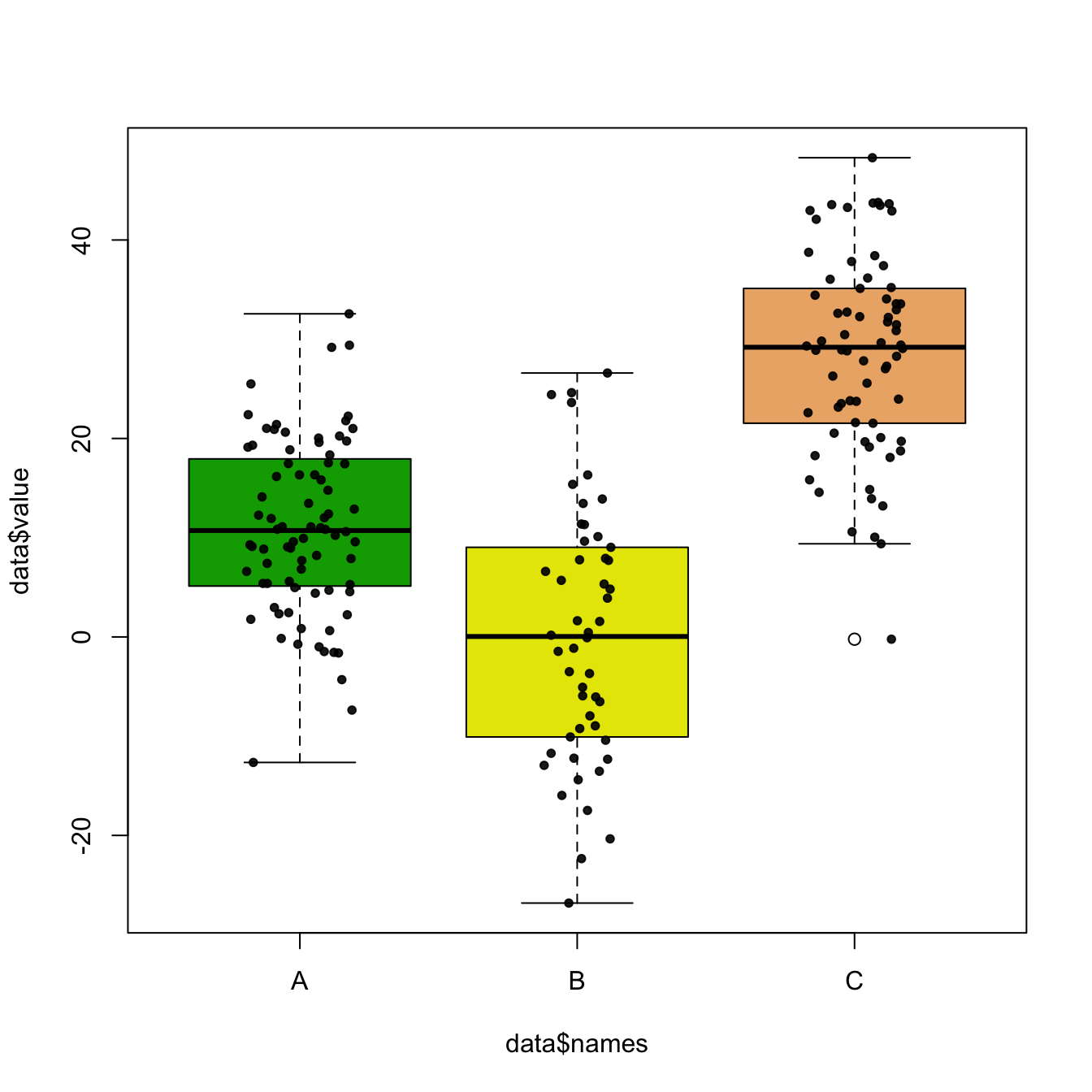# Boxplot with jitter in base R

Boxplot hides the distribution behind each group. This post show how to tackle this issue in base R, adding individual observation using dots with jittering.

Boxplot can be dangerous: the exact distribution of each group is hidden behind boxes as explained in data-to-viz.

If the amount of observation is not too high, you can add individual observations on top of boxes, using jittering to avoid dot overlap.

In base R, it is done manually creating a function that adds dot one by one, computing a random X position for all of them.``````# Create data
names <- c(rep("A", 80) , rep("B", 50) , rep("C", 70))
value <- c( rnorm(80 , mean=10 , sd=9) , rnorm(50 , mean=2 , sd=15) , rnorm(70 , mean=30 , sd=10) )
data <- data.frame(names,value)

# Basic boxplot
boxplot(data\$value ~ data\$names , col=terrain.colors(4) )

mylevels <- levels(data\$names)
levelProportions <- summary(data\$names)/nrow(data)
for(i in 1:length(mylevels)){

thislevel <- mylevels[i]
thisvalues <- data[data\$names==thislevel, "value"]

# take the x-axis indices and add a jitter, proportional to the N in each level
myjitter <- jitter(rep(i, length(thisvalues)), amount=levelProportions[i]/2)
points(myjitter, thisvalues, pch=20, col=rgb(0,0,0,.9))

}``````

Related chart types

## Contact

This document is a work by Yan Holtz. Any feedback is highly encouraged. You can fill an issue on Github, drop me a message on Twitter, or send an email pasting yan.holtz.data with gmail.com.